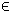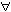In `mipq_prob` there are 4 mixed-integer quadratic programming test problems with sizes to about 120 variables and slightly more than 100 constraints. In order to define the problem `n` and solve it execute the following in Matlab:
```  Prob   = probInit('miqp_prob',n);
Result = tomRun('',Prob);
```
The basic structure of a general mixed-integer quadratic programming problem is:

 min x
f(x) =
1
2
xT F x + cT x

s/t
 xL ≤ x ≤ xU, bL ≤ A x ≤ bU,  xjNjI
(6)
where c, x, xL, xURn, ARm1 × n, and bL,bURm1. The variables xI, the index subset of 1,...,n are restricted to be integers.

An example of a problem of this class, (that is also found in the TOMLAB quickguide) is mipqQG:

File: tomlab/quickguide/miqpQG.m
```% miqpQG is a small example problem for defining and solving
% mixed-integer quadratic programming problems using the TOMLAB format.

c    = [-6 0]';
Name = 'XP Ref Manual MIQP';
F    = [4 -2;-2 4];
A    = [1 1];
b_L  = -Inf;
b_U  = 1.9;
x_L  = [0 0]';
x_U  = [Inf Inf]';

% Defining first variable as an integer
IntVars   = 1;

% Assign routine for defining a MIQP problem.
Prob = miqpAssign(F, c, A, b_L, b_U, x_L, x_U, [], ...
IntVars, [], [], [], Name, [], []);

% Calling driver routine tomRun to run the solver.
% The 1 sets the print level after optimization.

Result = tomRun('cplex', Prob, 1);
%Result = tomRun('oqnlp', Prob, 1);
%Result = tomRun('miqpBB', Prob, 1);
%Result = tomRun('xpress-mp', Prob, 1);
%Result = tomRun('minlpBB', Prob, 1);```